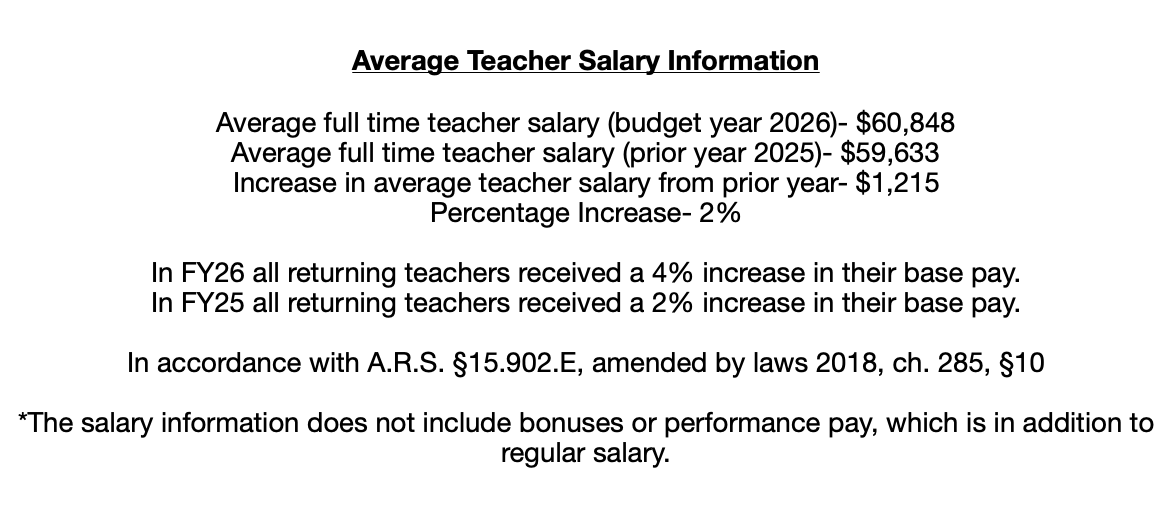# Math

HS Math Classes

Algebra:

• One subject, college ruled composition notebook or loose-leaf paper for note-taking.
• Quarter (1/4) inch graphing paper
• Ruler
• Graphing calculator, TI-83 or TI-84 preferred.

Physics:

• One subject, college ruled composition notebook or loose-leaf paper for note-taking.
• Composition notebook to be used as a Lab Notebook.
• Standard calculator (graphing calculator preferred, TI-83 or TI-84)

Chemistry

• One subject, college ruled composition notebook or loose-leaf paper for note-taking.
• Composition notebook to be used as a Lab Notebook.
• Standard calculator (graphing calculator preferred, TI-83 or TI-84)

Algebra II

• One subject, college ruled spiral-bound notebook for taking notes.
• Loose-leaf lined paper and quarter-inch graph paper.
• A working writing utensil.
• A standard Texas Instruments graphing calculator (TI-82, TI-83, TI-84, or TI-89). No cell phones, iPads, tablets, or iPods can be used as a calculator.

Geometry

• One subject, college ruled spiral-bound notebook for taking notes.
• Loose-leaf college ruled paper
• Quarter (1/4) inch graphing paper
• A working writing utensil.
• A standard Texas Instruments graphing calculator (TI-82, TI-83, TI-84, or TI-89). No cell phones, iPads, tablets, or iPods can be used as a calculator.
• A compass, protractor, and ruler.

PreCalculus

• One subject, college ruled spiral-bound notebook for taking notes.
• Loose-leaf lined paper and quarter-inch graph paper.
• A working writing utensil.
• A standard Texas Instruments graphing calculator (TI-82, TI-83, TI-84, or TI-89). No cell phones, iPads, tablets, or iPods can be used as a calculator.ASBCS Performance Dashboard